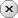### 用户注册### 用户登录### 发表随想Python自学    -  云代码空间

—— python自学——怎么学习python,自学python要多久？python+人工智能+人工智能+人工智能+大数据分析

# Python实战 | 如何使用Python开发高效的推荐算法？

2023-07-12|172阅||

## 推荐算法的分类

• 基于内容的推荐算法：该算法根据物品的属性和用户的历史行为，向用户推荐与其过去喜欢的物品相似的物品。

• 协同过滤推荐算法：该算法根据用户的历史行为和其他用户的行为，向用户推荐其他用户喜欢的物品。

• 混合推荐算法：该算法结合了基于内容的推荐算法和协同过滤推荐算法的优点，综合考虑物品本身的属性和用户之间的相似度，向用户推荐个性化的内容。

## 基于Python的推荐算法开发

### 数据集的准备

```import pandas as pd

# 读取数据集

ratings.csv

userId movieId rating
1 1 4.0
1 3 3.0
2 1 3.5
3 1 5.0
3 2 2.5

movies.csv

movieId title genres
3 Grumpier Old Men (1995) Comedy
4 Waiting to Exhale (1995) Comedy
5 Father of the Bride Part II (1995) Comedy

### 数据集的处理

```import numpy as np

# 转换数据集为用户-物品评分矩阵
ratings_matrix = ratings.pivot(index='userId', columns='movieId', values='rating').fillna(0).values```

#### 计算物品之间的相似度

```from sklearn.metrics.pairwise import cosine_similarity

# 计算电影之间的相似度矩阵
item_similarities = cosine_similarity(ratings_matrix.T)```

`推荐电影`

```# 定义推荐函数
def recommend_movies(user_id, ratings_matrix, item_similarities, N=10):
# 获取用户对电影的评分
user_ratings = ratings_matrix[user_id, :]
# 获取用户未评分的电影
unrated_movies = np.where(user_ratings == 0)
# 计算用户对未评分电影的评分预测值
scores = np.dot(item_similarities, user_ratings) / np.sum(item_similarities, axis=1)
# 对预测值排序，推荐Top N电影
top_movies = np.argsort(scores)[::-1][:N]
# 返回推荐的电影

# 使用示例
user_id = 0
top_movies = recommend_movies(user_id, ratings_matrix, item_similarities, N=10)
print(top_movies)```

### 实战

```from sklearn.metrics import mean_squared_error

# 随机选择一个用户
user_id = np.random.choice(ratings['userId'].unique())

# 将用户历史评分中20%的电影作为测试集
user_ratings = ratings[ratings['userId'] == user_id]
test_ratings = user_ratings.sample(frac=0.2, random_state=42)
train_ratings = user_ratings.drop(test_ratings.index)

# 构建推荐模型
train_matrix = train_ratings.pivot(index='userId', columns='movieId', values='rating').fillna(0).values
item_similarities = cosine_similarity(train_matrix.T)

# 评估推荐模型性能
test_matrix = test_ratings.pivot(index='userId', columns='movieId', values='rating').fillna(0).values
predicted_matrix = np.dot(item_similarities, train_matrix) / np.sum(item_similarities, axis=1).reshape(-1, 1)
rmse = np.sqrt(mean_squared_error(test_matrix[test_matrix.nonzero()], predicted_matrix[test_matrix.nonzero()]))

print(f"用户{user_id}的推荐模型RMSE为{rmse:.4f}")```

发表评论

## 个人资料

• 昵称： Python自学
• 等级： 高级设计师
• 积分： 5710
• 代码： 479 个
• 文章： 27 篇
• 随想： 4 条
• 访问： 111 次
• 关注